# Volumetric Light

Volumetric light is the effect you see in a hazy air, when the light rays becomes visible because of light scattering which occurs due to mist, fog, dust etc.

If used carefully it can give much realism to a scene... or kill it.

The volumetric light in Blender can be generated only for Spot lights, once the 'Halo' button (Figure 18) is pressed.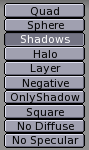Figure 18. Spot Light halo button.

If a test set up (Figure 19) is created, and the Halo button pressed the rendering will be like in Figure 19.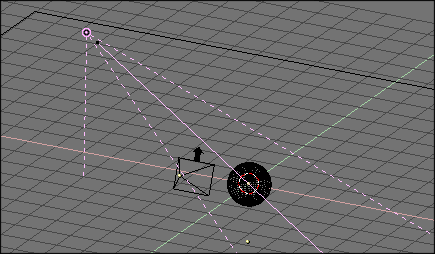Figure 19. Spot Light setup.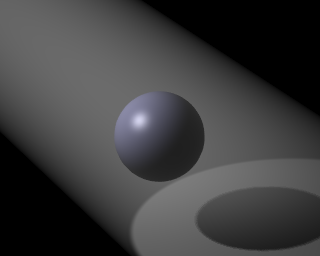Figure 20. Halo rendering.

The volumetric light effect is rather strong. The intensity of the Halo can be regulated with the HaloInt slider (Figure 21). Lower values corresponding to weaker halos.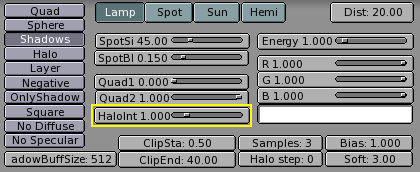Figure 21. Halo Intensity Slider.

The result is interesting. We have volumetric light, but we lack volumetric shadow! The halo passes through the sphere, yet a shadow is cast. This is due to the fact that the Halo occurs in the whole Spot Light cone unless we tell Blender to do otherwise.

The cone needs to be sampled to get volumetric shadow, and the sampling occurs with a step defined by the HaloStep NumButton (Figure 22). The default value of 0 means no sampling at all, hence the lack of volumetric shadow. A value of 1 gives finer stepping, and hence better results, but with a slower rendering time (Figure 23) while a higher value gives worse results but with faster renderings (Figure 24).Figure 22. Halo Step NumButton.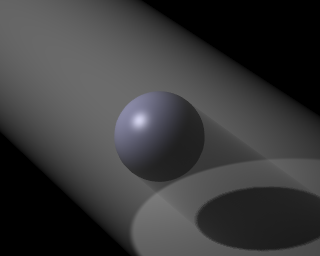Figure 23. Halo with volumetric shadow, Halo Step = 1.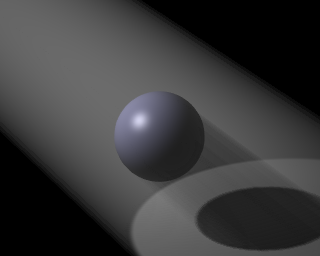Figure 24. Halo with volumetric shadow, Halo Step = 12.

 HaloStep valuesA value of 8 is usually a good compromise between speed and accuracy